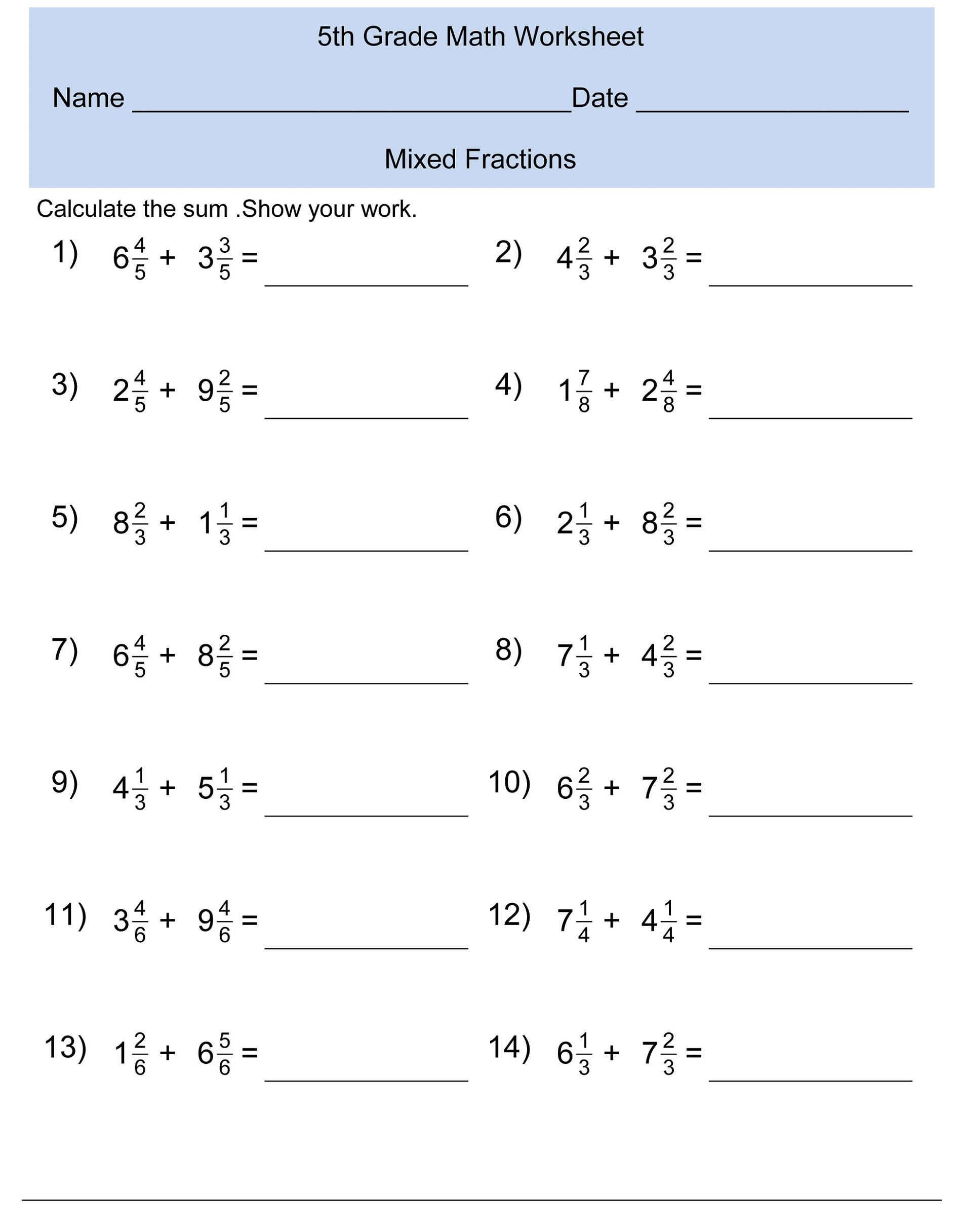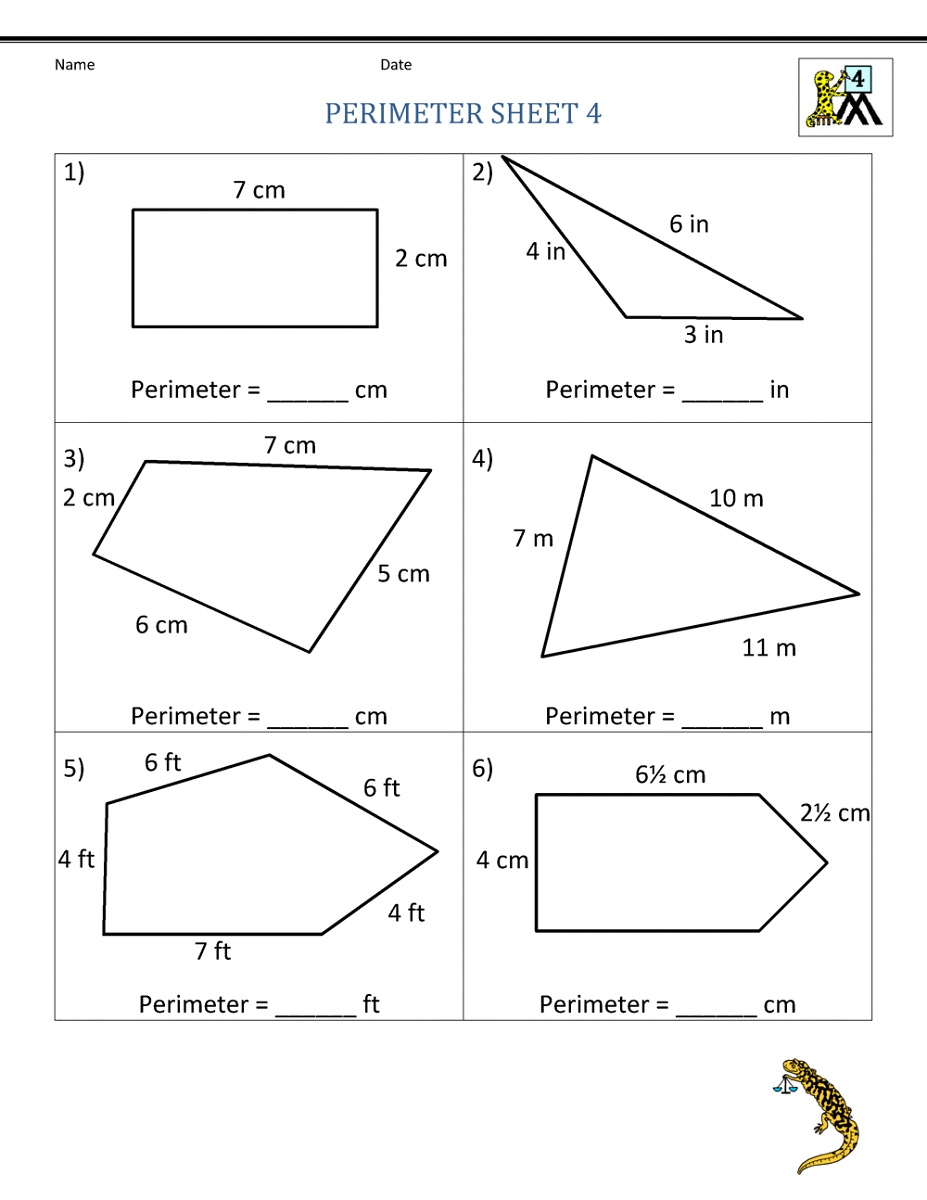#### Tagmath

The first feeling of mathematics is very essential. When learners think that studying mathematics is simple and enjoyable, it will be light for them to learn fundamental mathematical ideas at a subsequent point. It is also owing to the pupils ‘ proposal that mathematical teaching is enjoyable with the original feeling of pleasant teaching in mathematics. As a good learner, you may need good worksheets such as Grade 5 Math Worksheets Printable.Given the significance of presenting fundamental ideas, learners who decide to learn more should be introduced to mathematics. In this case, the teacher is a facilitator and a guide to the mathematical ideas of his students. They need the best facility such as Grade 4 Math Worksheets to Print.Provided in this page are printable math worksheets to use as a review tool on important math skills.

This Math Practice Worksheets include exercise such as, subtraction, addition, algebra, and more. These worksheets will be really effective to use both in classroom or home.

Here is our Time Tables 1-12 page where you will find printable times tables charts from 1 to 12. Multiplication charts can be used when students are having challenges memorizing math facts and/or understanding basic math concepts such as multiplication.

Your kids should know how addition and subtraction work together and they can study that by examining the relationship between numbers. Practice their number relationship by working on printable family of facts worksheets that we will share in this page.

Follow the list of fact family worksheets below to get all you need!

Free and printable 23 Times Table to help your child develop their multiplying skill! Advanced students can improve their times table skills by practicing with these by 23 multiplication charts.

Explore our entire collection of multiplication charts and find worksheet on by 23 multiplication!

Boost your students’ learning with this collection of educational and helpful Free Printable Math Workbooks. This collection of math worksheets is a great activity to teach your children or students as a fun way to reinforce important math skills.

Browse our collection of workbooks in the list provided below.